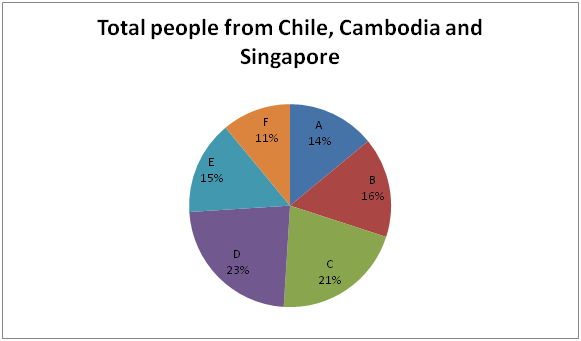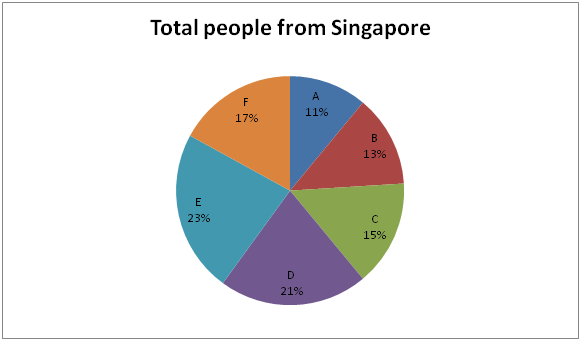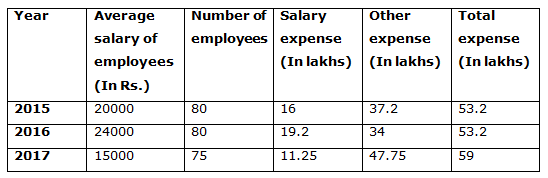# Quantitative Aptitude Questions (Data Interpretation) for Indian Bank PO Mains 2018 Day-213

Dear Readers, Indian Bank is conducting Online Examination for the recruitment of PO. To enrich your preparation here we have providing new series of Data Interpretation – Quantitative Aptitude Questions. Candidates those who are appearing in Indian Bank PO Mains Exams can practice these Quantitative Aptitude average questions daily and make your preparation effective.

[WpProQuiz 3914]

Directions (Q. 1 – 5) Study the following information carefully and answer the given questions:

In the year 2015, the salary expense of a company is 16 lakhs and the total number of employees is 80 and in the year 2016, the average salary of employees is Rs. 4000 more than the previous year. The salary expense in 2016 is 20 % more than the previous year. The other expense in the year 2016 is 34 lakhs. The total expense in the year 2015 and 2016 is same. In the year 2017, the number of employees is 5 less than the previous year. The average salary of employees in the year 2017 is Rs. 15000 and the other expense in the year 2017 is Rs. 47.75 lakhs.

Note:

Salary expense = Average salary of employees * Number of employees

Total expense = Salary expense + other expense

1) The total expense in the year 2015 is approximately what percentage of total expense in the year 2017?

a) 110 %

b) 100 %

c) 75 %

d) 90 %

e) 60 %

2) Find the total average salary of employees in all the given years together?

a) Rs. 59000

b) Rs. 48000

c) Rs. 53000

d) Rs. 56000

e) None of these

3) Find the difference between the number of employees in the year 2015 to that in the year 2017?

a) 10

b) 15

c) 5

d) 12

e) None of these

4) Find the average other expense in all the given years together?

a) 39.65 lakhs

b) 35.72 lakhs

c) 33.58 lakhs

d) 41.25 lakhs

e) None of these

5) The total expense in the year 2017 is approximately what percentage more/less than the other expense in the year 2016?

a) 50 % more

b) 60 % less

c) 74 % more

d) 50 % less

e) 74 % less

Directions (Q. 6 – 10) Study the following information carefully and answer the given questions:

The following pie chart1 shows the percentage distribution of total number of peoples from Chile, Cambodia and Singapore went for an art exhibition from different cities.

Total peoples = 80000The pie chart2 shows the percentage distribution of total number of people from Singapore went for the same exhibition from different cities.

Total peoples = 300006) Find the difference between the total number of Chile and Cambodia peoples went for an art exhibition from city B to that in city E?

a) 2700

b) 1900

c) 3800

d) 2400

e) None of these

7) Find the ratio between the total number of people went for an art exhibition from Chile and Cambodia in city C to that of total number of people went for an art exhibition from Singapore in city D?

a) 41: 21

b) 32: 17

c) 25: 11

d) 57: 23

e) None of these

8) The total number of people went for an art exhibition from city A in all the given countries together is approximately what percentage of total number of people went for an art exhibition from Singapore in city B?

a) 245 %

b) 230 %

c) 198 %

d) 287 %

e) 182 %

9) Find the central angle of total number of people went for an art exhibition from all the given countries in city E?

a) 480

b) 540

c) 660

d) 720

e) None of these

10) If the total number of male to that of female went for an art exhibition from Chile and Cambodia in city C is 2: 1, then find the number of male went for an art exhibition from Chile and Cambodia in city C?

a) 7800

b) 9500

c) 8200

d) 6600

e) None of these

Directions (1-5):

In the year 2015,

The salary expense of a company = 16 lakhs

The total number of employees = 80

Average salary of employees = 1600000/80 = Rs. 20000

In the year 2016,

The average salary of employees = Rs. 1000 + Average salary of employees in 2015

= > 4000 + 20000 = Rs. 24000

The salary expense in 2016 = (120/100)*16 = 19.2 lakhs

The other expense in the year 2016 = 34 lakhs

Total expense in the year 2016 = 19.2 + 34 = 53.2 lakhs

The number of employees in 2015 = 1920000/24000 = 80

The total expense in the year 2015 and 2016 is same. So,

The total expense in the year 2015 = 53.2 lakhs

The other expense in the year 2015 = 53.2 – 16 = 37.2 lakhs

The average salary of employees in the year 2017 = Rs. 15000

The other expense in the year 2017 = Rs. 47.75 lakhs

The number of employees in 2017 = 80 – 5 = 75

The salary expense in 2017 = 75*15000 = 11.25 lakhs

The total expense in the year 2017 = 47.75 + 11.25 = 59 lakhsThe total expense in the year 2015 = 53.2 lakhs

The total expense in the year 2017 = 59 lakhs

Required % = (53.2/59)*100 = 90 %

Explanation:

The total average salary of employees in all the given years together

= > 20000 + 24000 + 15000 = Rs. 59000

Explanation:

The number of employees in the year 2015 = 80

The number of employees in the year 2017 = 75

Required difference = 80 – 75 = 5

Explanation:

The average salary expense in all the given years together

= > (37.2 + 34 + 47.75)/3

= > 118.95/3 = 39.65 lakhs

Explanation:

The total expense in the year 2017 = 59

The other expense in the year 2016 = 34

Required % = [(59 – 34)/34]*100 = 74 % more

Direction (6-10) :

Explanation:

The total number of Chile and Cambodia peoples went for an art exhibition from city B

= > 80000*(16/100) – 30000*(13/100)

= > 12800 – 3900 = 8900

The total number of Chile and Cambodia peoples went for an art exhibition from city E

= > 80000*(15/100) – 30000*(23/100)

= > 12000 – 6900 = 5100

Required difference = 8900 – 5100 = 3800

Explanation:

The total number of people went for an art exhibition from Chile and Cambodia in city C

= > 80000*(21/100) – 30000*(15/100)

= > 16800 – 4500 = 12300

The total number of people went for an art exhibition from Singapore in city D

= > 30000*(21/100) = 6300

Required ratio = 12300: 6300 = 41: 21

Explanation:

The total number of people went for an art exhibition from city A in all the given countries together

= > 80000*(14/100)

The total number of people went for an art exhibition from Singapore in city B

= > 30000*(13/100)

Required % = {[80000*(14/100)]/[ 30000*(13/100]}*100 = 287 %

Explanation:

The total number of people went for an art exhibition from Chile and Cambodia in city C

= > 80000*(21/100) – 30000*(15/100)

= > 16800 – 4500 = 12300

The total number of male to that of female went for an art exhibition from Chile and Cambodia in city C = 2: 1

Number of male went for an art exhibition from Chile and Cambodia in city C

= > (2/3)*12300 = 8200

Daily Practice Test Schedule | Good Luck

 Topic Daily Publishing Time Daily News Papers & Editorials 8.00 AM Current Affairs Quiz 9.00 AM Quantitative Aptitude “20-20” 11.00 AM Vocabulary (Based on The Hindu) 12.00 PM General Awareness “20-20” 1.00 PM English Language “20-20” 2.00 PM Reasoning Puzzles & Seating 4.00 PM Daily Current Affairs Updates 5.00 PM Data Interpretation / Application Sums (Topic Wise) 6.00 PM Reasoning Ability “20-20” 7.00 PM English Language (New Pattern Questions) 8.00 PM